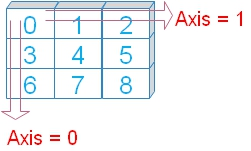# append() : Adding to Array

We can add values at the end of a Numpy array by using append().
``numpy.append(arr, values, axis=None)``
`arr` : Values are added to the copy of this array.
`values` : Values to be added to arr. Must be of same shape of the arr ( if Axis is present ) . If Axis is not specified then matching shape of arr is not prerequisite.
`axis` : (Optional) Direction in which the values to be appended.

Note that there is no change to the original array.
``````import numpy as np
npr=np.array([5,8,3])
npr1=np.append(npr,10)
print(npr) # [5 8 3]  , No change to original array
print(npr1)# [ 5  8  3 10], element added at the end``````

## Using 2-D array

``````import numpy as np
npr=np.array([[0,1,2,4],[3,4,5,6],[6,7,8,9]]) #
print(npr.ndim) # 2
print(npr.shape) # (3,4)
print(npr)
npr1=np.append(npr,[[10,11,12,13]],axis=0)
print(npr1)``````
Output
``````2
(3, 4)
[[0 1 2 4]
[3 4 5 6]
[6 7 8 9]]
[[ 0  1  2  4]
[ 3  4  5  6]
[ 6  7  8  9]
[10 11 12 13]]``````
We must take care that while adding we have the same dimension along the axis of addition. For any mis-match we will get error like this.
ValueError: all the input arrays must have same number of dimensions

## Appending using axisAxis are the directions in rows and columns.

Axis 0 is the downward direction or in rows order.
Axis 1 is the horizontal direction or in column order.
``````import numpy as np
npr=np.array([[0,1,2,4],[3,4,5,6],[6,7,8,9]])
npr1=np.array([[10,11,12,13],[21,22,23,24],[31,32,34,35]])
npr2=np.append(npr,npr1,axis=0)
print(npr2)``````
Output
``````[[ 0  1  2  4]
[ 3  4  5  6]
[ 6  7  8  9]
[10 11 12 13]
[21 22 23 24]
[31 32 34 35]]``````
Change the Axis to 1
``npr2=np.append(npr,npr1,axis=1)``
Output
``````[[ 0  1  2  4 10 11 12 13]
[ 3  4  5  6 21 22 23 24]
[ 6  7  8  9 31 32 34 35]]``````
By using reshape() we can match the requirements of append ( to match the dimensions ) and then use.
``````import numpy as np
npr=np.array([[0,1,2,4],[3,4,5,6],[6,7,8,9]])
npr1=np.array([10,11,12,13,21,22,23,24,31,32,34,35]) # different shape
#npr1=npr1.reshape(3,4)
npr1=npr1.reshape(npr.shape) # Match the shape of first array
npr2=np.append(npr,npr1,axis=1)
print(npr2)``````
Output
``````[[ 0  1  2  4 10 11 12 13]
[ 3  4  5  6 21 22 23 24]
[ 6  7  8  9 31 32 34 35]]``````

## Example

Adding one (or two )element at the end of each row. Note how the shape is matched.
``````import numpy as np
npr=np.array([[0,1,2,4],[3,4,5,6],[6,7,8,9]])
npr1=np.array([,,]) # One element for each row
#npr1=np.array([[10,5],[11,6],[12,7]]) # Two elements for each row
npr2=np.append(npr,npr1,axis=1)
print(npr2)``````
Output
``````[[ 0  1  2  4 10]
[ 3  4  5  6 11]
[ 6  7  8  9 12]]``````
If axis is not specified then arrays are flattened before adding. We can use any dimension array and shape of the arrays is not a prerequisite here.
``````import numpy as np
npr=np.array([[0,1,2,4],[3,4,5,6],[6,7,8,9]])
npr1=np.array([[10,11],[12,13]])
npr2=np.append(npr,npr1)
print(npr2) # [ 0  1  2  4  3  4  5  6  6  7  8  9 10 11 12 13]``````

Subscribe to our YouTube Channel here

## Subscribe

* indicates required
Subscribe to plus2netplus2net.com